# 置顶JavaScript 函数式编程（二）精选热门

slide 地址

## 三、可以，这很函数式~### 3.1.函数是一等公民！

#### 3.1.1.滥用匿名函数

``````// 太傻了
const getServerStuff = function (callback) {
return ajaxCall(function (json) {
return callback(json)
})
}

// 这才像样
const getServerStuff = ajaxCall

// 下面来推导一下...
const getServerStuff
=== callback => ajaxCall(json => callback(json))
=== callback => ajaxCall(callback)
=== ajaxCall

// from JS函数式编程指南
``````

``````const BlogController = (function () {
const index = function (posts) {
return Views.index(posts)
}

const show = function (post) {
return Views.show(post)
}

const create = function (attrs) {
return Db.create(attrs)
}

const update = function (post, attrs) {
return Db.update(post, attrs)
}

const destroy = function (post) {
return Db.destroy(post)
}

return { index, show, create, update, destroy }
})()

// 以上代码 99% 都是多余的...

const BlogController = {
index: Views.index,
show: Views.show,
create: Db.create,
update: Db.update,
destroy: Db.destroy,
}

// ...或者直接全部删掉
// 因为它的作用仅仅就是把视图（Views）和数据库（Db）打包在一起而已。

// from JS函数式编程指南
``````

#### 3.1.2.为何钟爱一等公民？

``````// 原始函数
httpGet('/post/2', function (json) {
return renderPost(json)
})

// 假如需要多传递一个 err 参数
httpGet('/post/2', function (json, err) {
return renderPost(json, err)
})

// renderPost 将会在 httpGet 中调用，
// 想要多少参数，想怎么改都行
httpGet('/post/2', renderPost)
``````

#### 3.1.3.提高函数复用率

``````// 只针对当前的博客
const validArticles = function (articles) {
return articles.filter(function (article) {
return article !== null && article !== undefined
})
}

// 通用性好太多
const compact = function(xs) {
return xs.filter(function (x) {
return x !== null && x !== undefined
})
}
``````

#### 3.1.4.this（江来报道上出了偏差…识得唔识得？）

### 3.2.柯里化（curry）

#### 3.2.1.柯里化概念

``````import { curry } from 'lodash'

const add = (x, y) => x + y

increment(2) // 3
``````

#### 3.2.2.柯里化 VS 偏函数应用（partial application）

In computer science, partial application (or partial function application) refers to the process of fixing a number of arguments to a function, producing another function of smaller arity.

by wikipedia

``````import { curry, partial } from 'lodash'

const add = (x, y, z) => x + y + z

``````

#### 3.2.3.柯里化的实现

``````// 实现一个函数 curry 满足以下调用、
const f = (a, b, c, d) => { ... }
const curried = curry(f)

curried(a, b, c, d)
curried(a, b, c)(d)
curried(a)(b, c, d)
curried(a, b)(c, d)
curried(a)(b, c)(d)
curried(a)(b)(c, d)
curried(a, b)(c)(d)
``````

``````// ES5
var curry = function curry (fn, arr) {
arr = arr || []

return function () {
var args = [].slice.call(arguments)
var arg = arr.concat(args)

return arg.length >= fn.length
? fn.apply(null, arg)
: curry(fn, arg)
}
}

// ES6
const curry = (fn, arr = []) => (...args) => (
arg => arg.length >= fn.length
? fn(...arg)
: curry(fn, arg)
)([...arr, ...args])
``````

#### 3.2.4.柯里化的意义

``````// 定义通用函数
const converter = (
toUnit,
factor,
offset = 0,
input
) => ([
((offset + input) * factor).toFixed(2),
toUnit,
].join(' '))

// 分别绑定不同参数
const milesToKm =
curry(converter)('km', 1.60936, undefined)
const poundsToKg =
curry(converter)('kg', 0.45460, undefined)
const farenheitToCelsius =
curry(converter)('degrees C', 0.5556, -32)

-- from https://stackoverflow.com/a/6861858
``````

``````function converter (ratio, symbol, input) {
return (input * ratio).toFixed(2) + ' ' + symbol
}

converter(2.2, 'lbs', 4)
converter(1.62, 'km', 34)
converter(1.98, 'US pints', 2.4)
converter(1.75, 'imperial pints', 2.4)

-- from https://stackoverflow.com/a/32379766
``````

### 3.3.函数组合（compose）

#### 3.3.1.组合的概念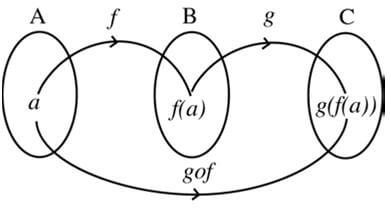#### 3.3.2.组合的实现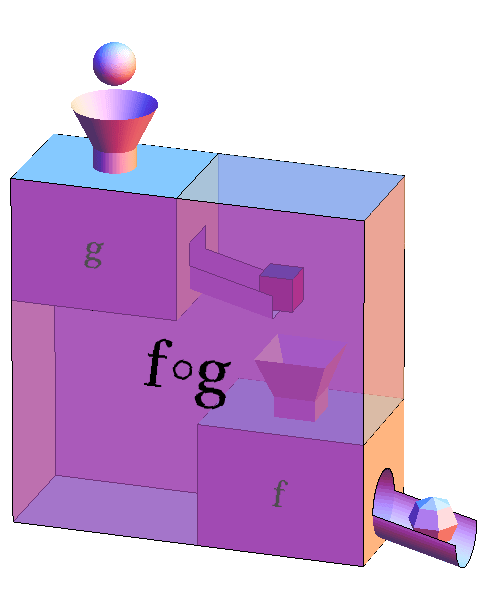``````const add1 = x => x + 1
const mul3 = x => x * 3
const div2 = x => x / 2

// redux 版
const compose = (...fns) => {
if (fns.length === 0) return arg => arg
if (fns.length === 1) return fns

return fns.reduce((a, b) => (...args) => a(b(...args)))
}

// 一行版，支持多参数，但必须至少传一个函数
const compose = (...fns) => fns.reduceRight((acc, fn) => (...args) => fn(acc(...args)))

// 一行版，只支持单参数，但支持不传函数
const compose = (...fns) => arg => fns.reduceRight((acc, fn) => fn(acc), arg)
``````

#### 3.3.3.Pointfree

Pointfree 即不使用所要处理的值，只合成运算过程。中文可以译作"无值"风格。

from Pointfree 编程风格指南

``````const addOne = x => x + 1
const square = x => x * x
``````

``````const addOneThenSquare = compose(square, addOne)
``````

``````// 非 Pointfree，因为提到了数据：word
const snakeCase = function (word) {
return word.toLowerCase().replace(/\s+/ig, '_')
}

// Pointfree
const snakeCase = compose(replace(/\s+/ig, '_'), toLowerCase)
``````

``````const square = n => n * n;

_.map([4, 8], square) // 第一个参数是待处理数据

R.map(square, [4, 8]) // 一般函数式库都将数据放在最后
``````

1.无法柯里化后偏函数应用

2.无法进行函数组合

3.无法扩展 map（reduce 等方法） 到各种其他类型

（详情参阅参考文献之《Hey Underscore, You’re Doing It Wrong!》）

#### 3.3.4.函数组合的意义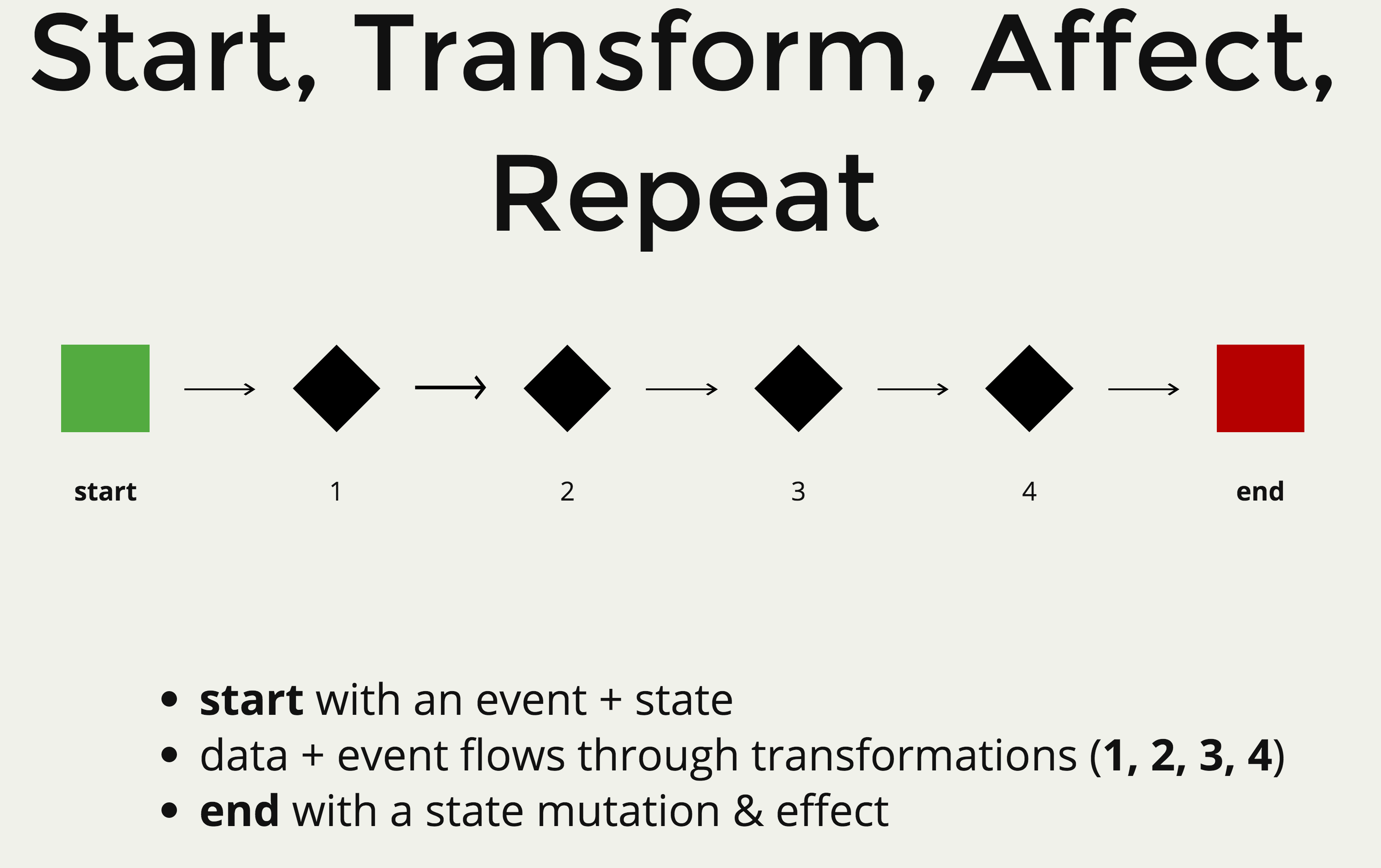• 一个 start 可以是：

• 开启应用
• 接收到的 HTTP 请求
• 返回的 HTTP 响应
• 查询数据库的结果
• WebSocket 消息
• 一个 end 或者说是 effect 可以是：

• 渲染或更新 UI
• 触发一个 DOM 事件
• 创建一个 HTTP 请求
• 返回一个 HTTP 响应
• 保存数据到 DB
• 发送 WebSocket 消息

• filter
• slice
• map
• reduce
• concat
• zip
• fork
• flatten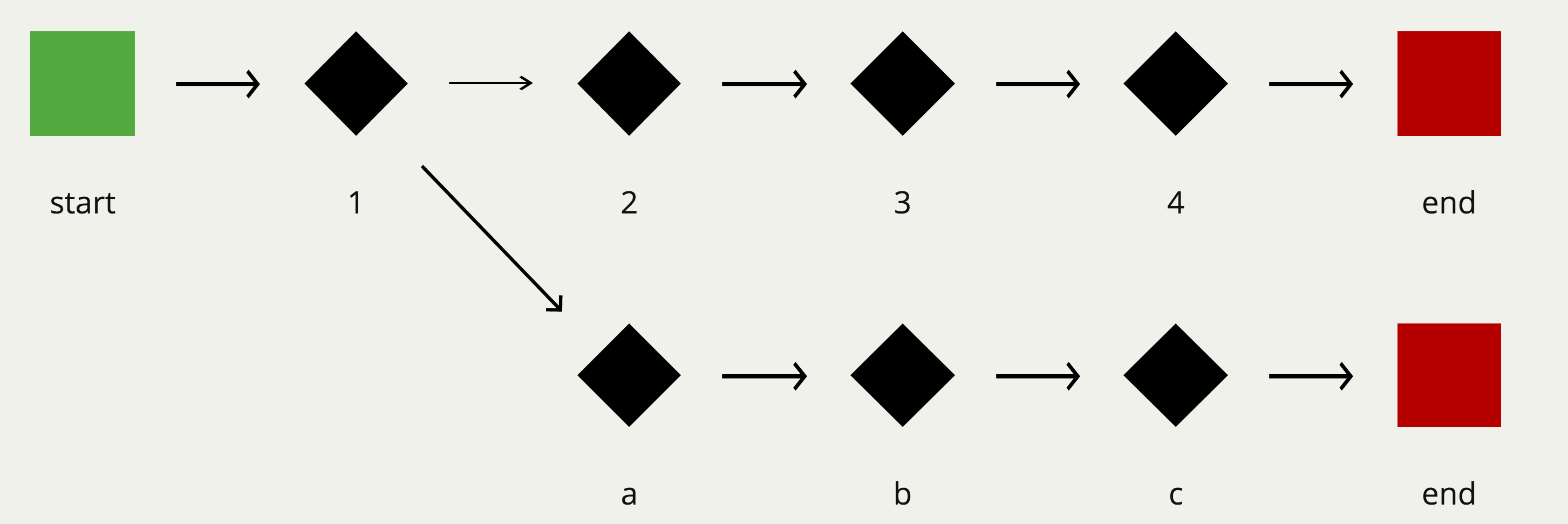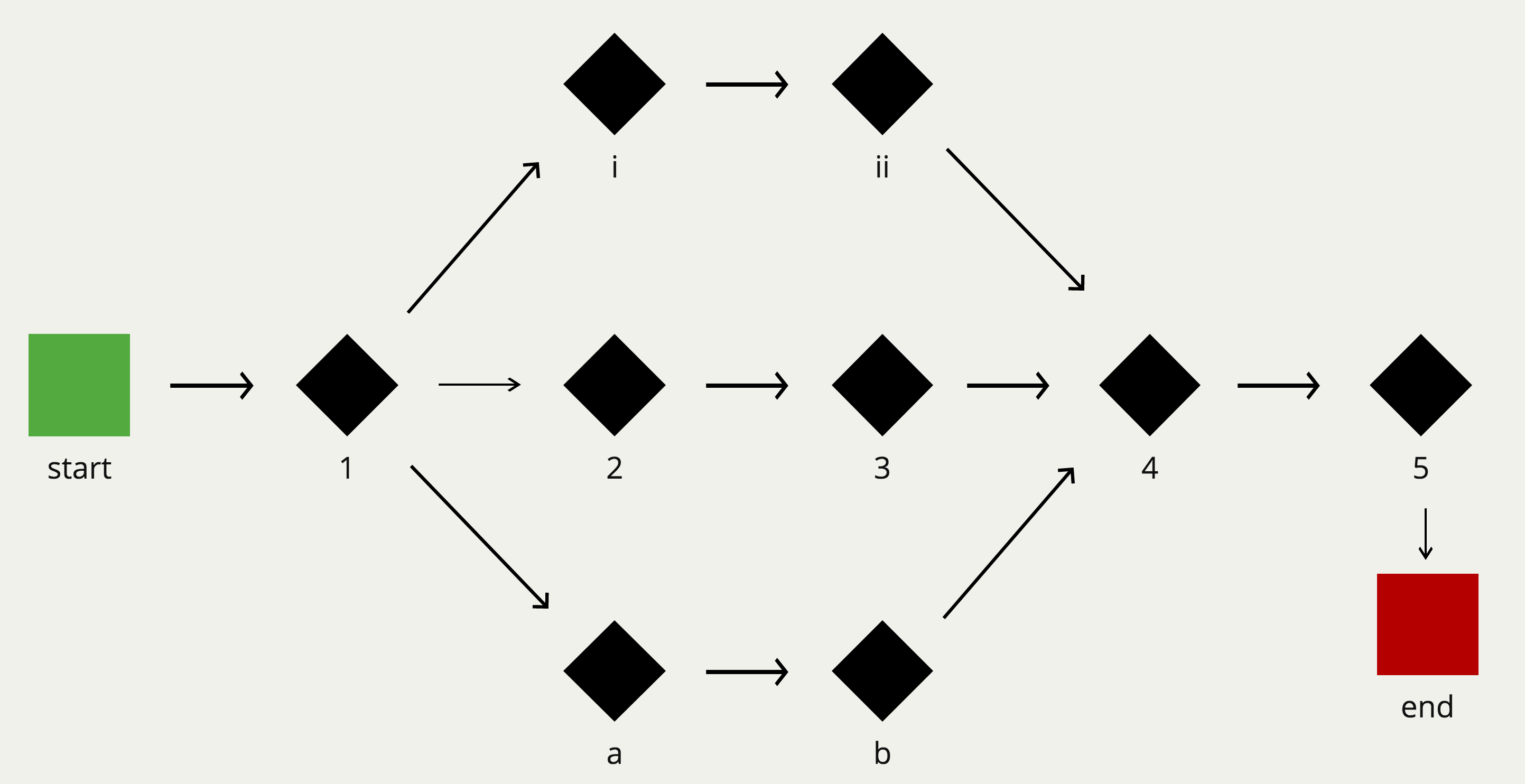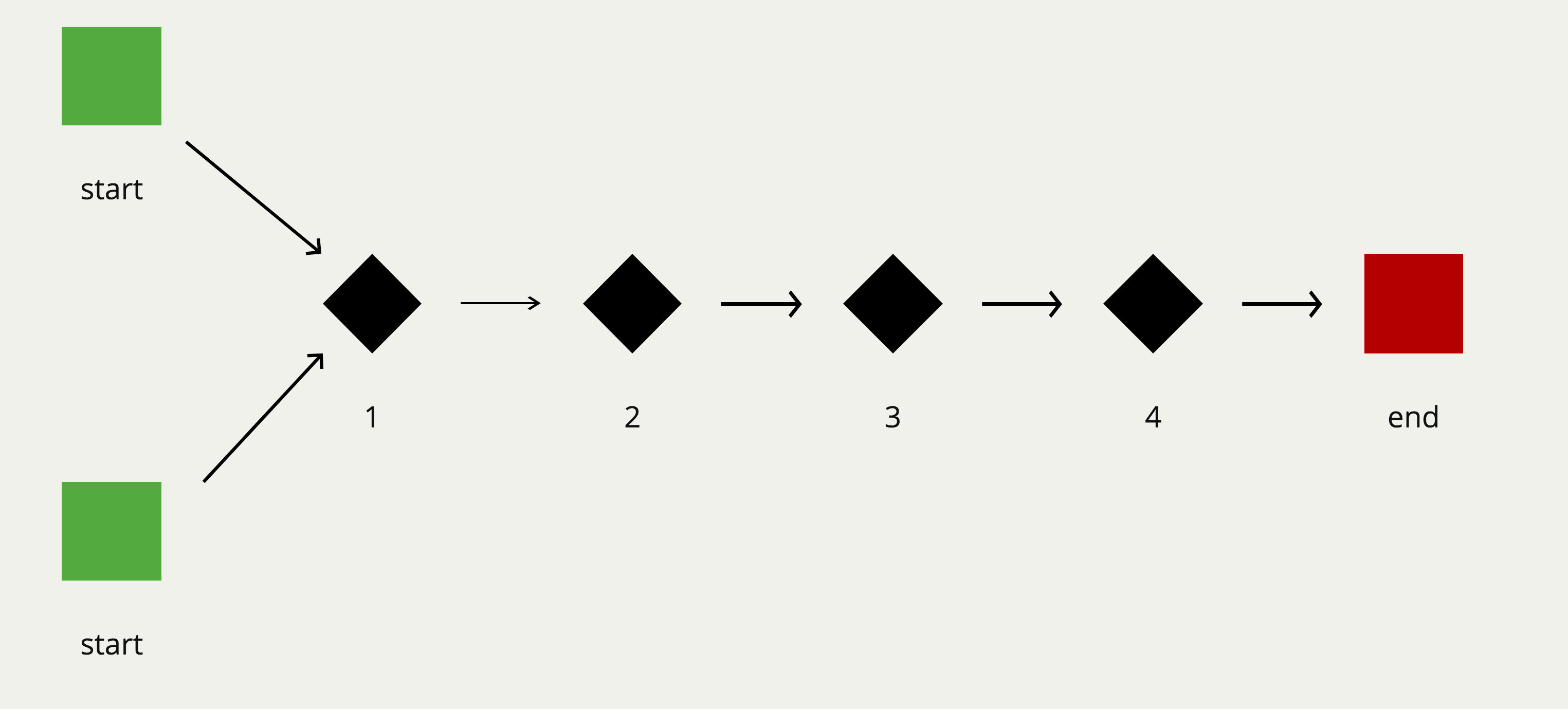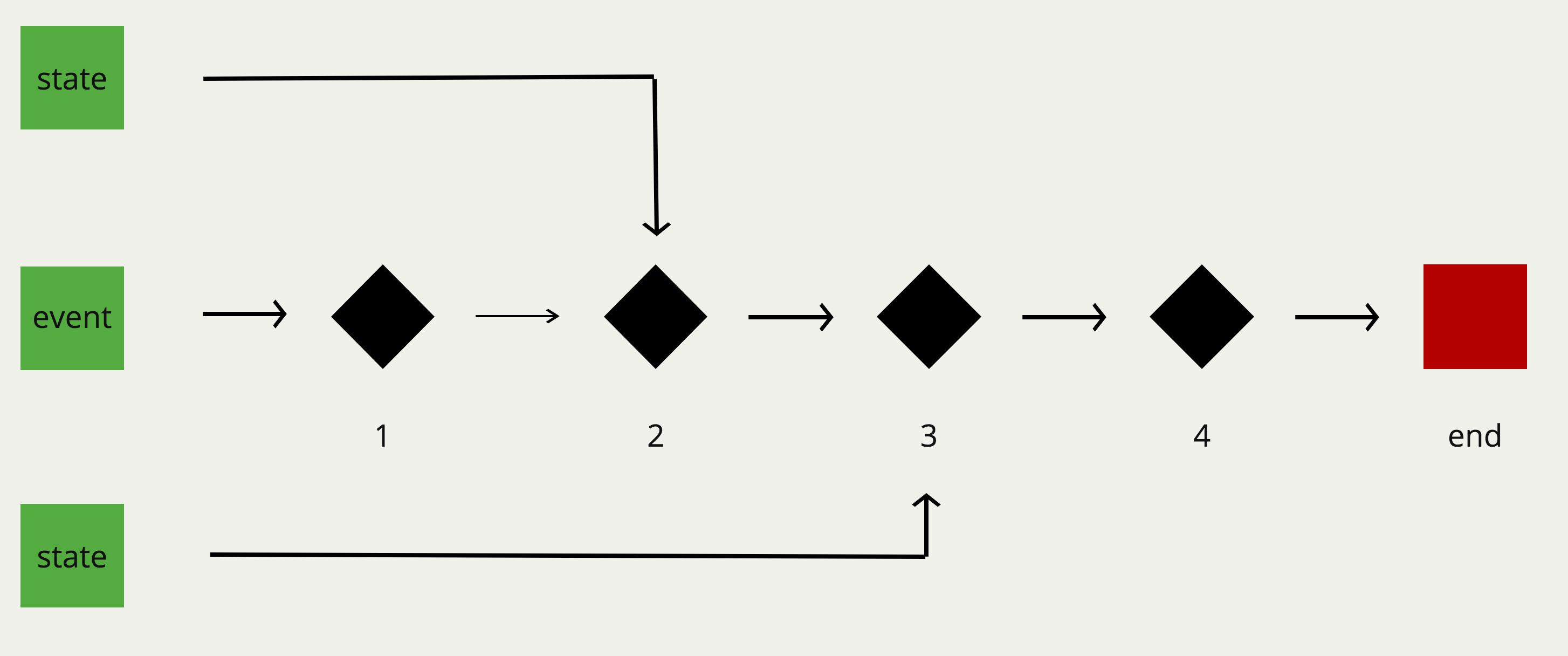### 3.4.Hindley-Milner 类型签名

#### 3.4.1.基本概念

``````// strLength :: String -> Number
const strLength = s => s.length

// join :: String -> [String] -> String
const join = curry((what, xs) => xs.join(what))

// match :: Regex -> String -> [String]
const match = curry((reg, s) => s.match(reg))

// replace :: Regex -> String -> String -> String
const replace = curry((reg, sub, s) => s.replace(reg, sub))
``````

``````// match :: Regex -> (String -> [String])
const match = curry((reg, s) => s.match(reg))
``````

``````// match :: Regex -> (String -> [String])
const match = curry((reg, s) => s.match(reg))

// onHoliday :: String -> [String]
const onHoliday = match(/holiday/ig)
``````

``````// replace :: Regex -> (String -> (String -> String))
const replace = curry((reg, sub, s) => s.replace(reg, sub))
``````

``````//  id :: a -> a
const id = x => x

//  map :: (a -> b) -> [a] -> [b]
const map = curry((f, xs) => xs.map(f))
``````

``````//  head :: [a] -> a
const head = xs => xs

//  filter :: (a -> Bool) -> [a] -> [a]
const filter = curry((f, xs) => xs.filter(f))

//  reduce :: (b -> a -> b) -> b -> [a] -> b
const reduce = curry((f, x, xs) => xs.reduce(f, x))
``````

reduce 可能是以上签名里让人印象最为深刻的一个，同时也是最复杂的一个了，所以如果你理解起来有困难的话，也不必气馁。为了满足你的好奇心，我还是试着解释一下吧；尽管我的解释远远不如你自己通过类型签名理解其含义来得有教益。

#### 3.4.2.参数态（Parametricity）

``````// head :: [a] -> a
``````

``````// reverse :: [a] -> [a]
``````

<img width=“200” src=“https://buptsteve.github.io/blog/imgs/fp-in-js/i-don’t-think-so.jpg” alt=“i-don’t-think-so”>

<img width=“200” src=“https://buptsteve.github.io/blog/imgs/fp-in-js/i-think-it-is-ok.jpg” alt=“i-think-it-is-ok”>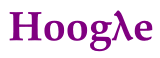#### 3.4.3.自由定理（Free Theorems）

``````// head :: [a] -> a

// filter :: (a -> Bool) -> [a] -> [a]
// 其中 f 和 p 是谓词函数
compose(map(f), filter(compose(p, f))) ===
compose(filter(p), map(f))
``````

#### 3.4.4.类型约束

``````// sort :: Ord a => [a] -> [a]
``````

Ord 到底是什么？它是从哪来的？在一门强类型语言中，它可能就是一个自定义的接口，能够让不同的值排序。通过这种方式，我们不仅能够获取关于 a 的更多信息，了解 sort 函数具体要干什么，而且还能限制函数的作用范围。我们把这种接口声明叫做类型约束（type constraints）。

``````// assertEqual :: (Eq a, Show a) => a -> a -> Assertion
``````

• 声明函数的输入和输出
• 让函数保持通用和抽象
• 可以用于编译时候检查
• 代码最好的文档

## 参考资料